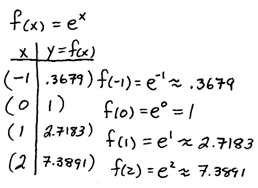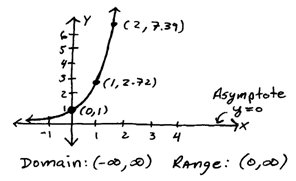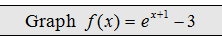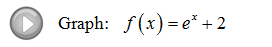## Pages

### The Natural Exponential Function

In this section we will introduce the natural base and define and graph the natural exponential function.
YouTube playlist for steps covering exponential functions and their graphsThis number is so special, just as is pi, that it has its own button on your scientific calculator. Try finding the e^x button on your calculator and using it to calculate e.

Evaluate.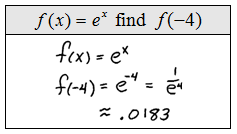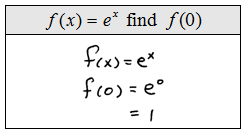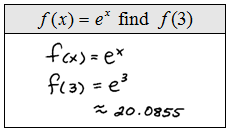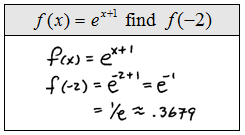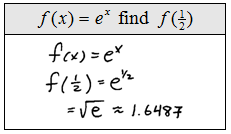Graph the following exponential functions. State the domain, range, and label the horizontal asymptote.